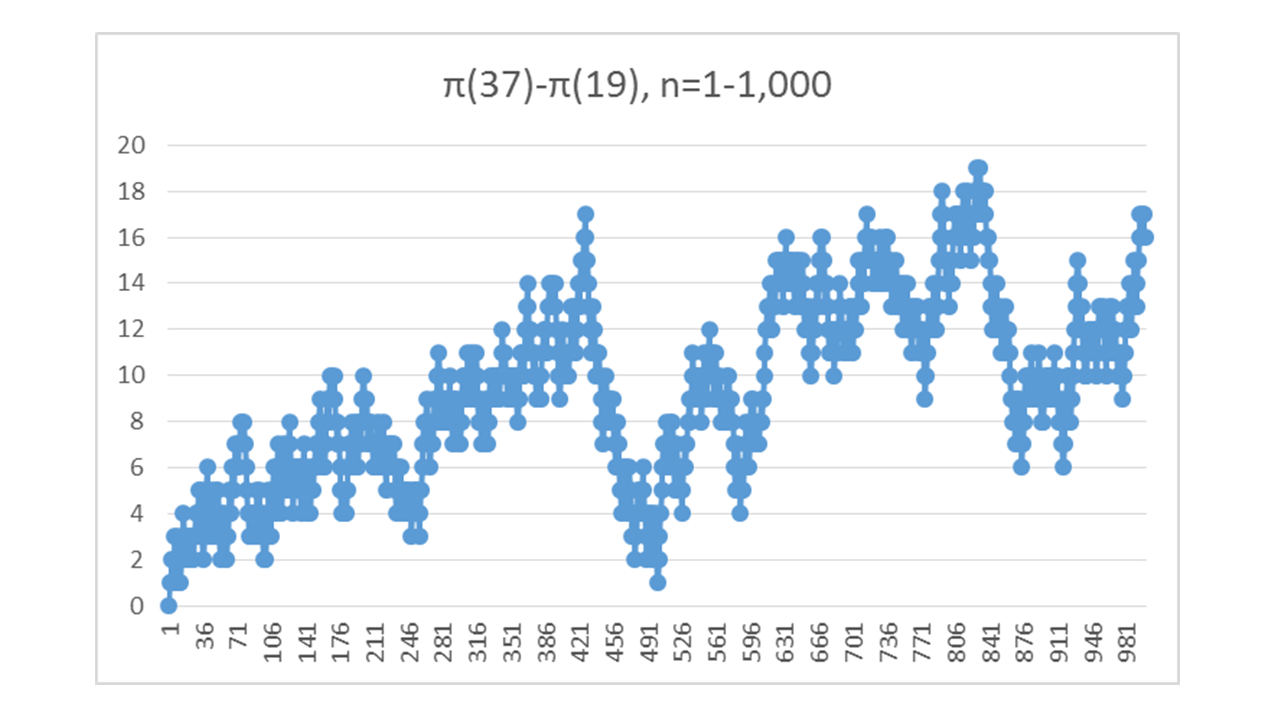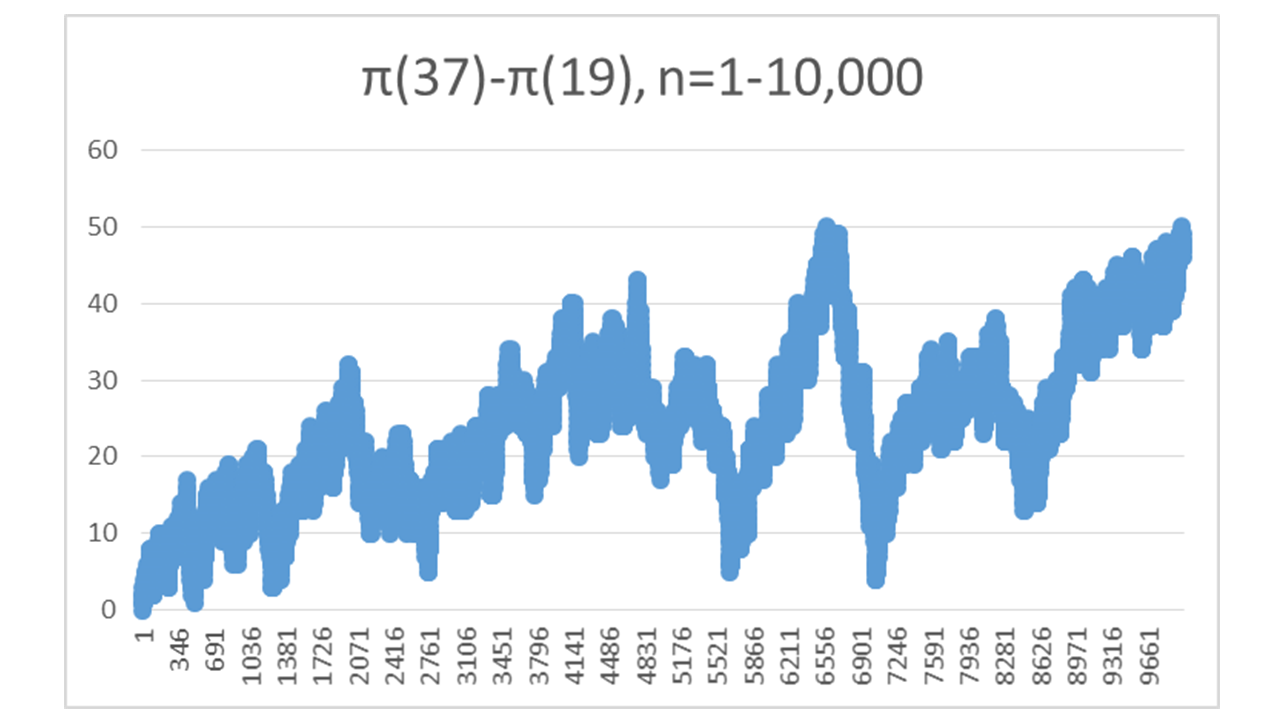Problems & Puzzles: Conjectures

Conjecture 73. Counting primes by the last significant digit.

Mr. Islem Ghaffor, sent the following observation and the corresponding conjecture.

For p>2, in base 10, the sum of the quantity of primes ending in 3 and 7 is always greater than the sum of the quantity of primes ending in 1 and 9.

Symbolically we may express this conjecture this way:

For p>2, π(3,7)-π(1,9)>0

Islem sent this conjecture after observing and counting the primes of each type less than 100. Currently he has not a proof of this conjecture.

Here is the table sent by Islem, where f(p(n))= π(3,7)-π(1,9). Here p(n) means the n-th prime number: p(1)=2, p(2)=3, and so on.

 n π(3,7) π(1,9) f(p(n)) 2 1 0 1 3 1 0 1 4 2 0 2 5 2 1 1 6 3 1 2 7 4 1 3 8 4 2 2 9 5 2 3 10 5 3 2 11 5 4 1 12 6 4 2 13 6 5 1
 14 7 5 2 15 8 5 3 16 9 5 4 17 9 6 3 18 9 7 2 19 10 7 3 20 10 8 2 21 11 8 3 22 11 9 2 23 12 9 3 24 12 10 2 25 13 10 3

Carlos Rivera tested and verified positively this conjecture for all the primes less than 2^30, computing and graphing also the function f(p(n))= π(3,7)-π(1,9) for two ranges.

Here you have two graphs for f(p(n)) a) for n=1 to 1,000 b) for n=1 to 10,000.Obviously the first point (n=1) is out of the conjecture and is the only point where π(3,7)-π(1,9)=0 instead of the range covered by the conjecture where π(3,7)-π(1,9)>0.

Q1. Would you like to continue verifying this conjecture?

Q2. Would you like to prove this conjecture?

As you may observe, it seems that f(p(n)) tends to grow, very slowly but it's seems to be growing without limit, but who knows...

Q3. What can you say about lim f(p(n)) as n goes to infinite?

Q4. What do you think about this conjecture, is it interesting/important or not, beyond a simple curio?

Contributions cam from J. K. Andersen, Giovanni Resta, Jean Brette and Luis Rodríguez

***

Andersen wrote:

The conjecture first fails at p(102091222) = 2082927019, where both counts are 51045610.
It was verified with two independent programs in C and PARI/GP.
It is known that pi(3,7)/pi(1,9) tends to 1.
I expect f(p(n)) to take any value infinitely many times.

Primes ending in each of the digits coprime to 10 has the same
asymptotic frequency. This holds for any base. See for example
http://primes.utm.edu/glossary/xpage/DirichletsTheorem.html

***

Resta wrote:

As it happens with similar conjectures
(see http://mathworld.wolfram.com/ChebyshevBias.html )
the conjecture is false.

At P = 2082927019  the counts t37=51045610 and t19=51045610
are equal and the difference is 0. Then it becomes negative
and then it changes sign many many times.

for example:

P= 2082927019 t37=51045610 t19=51045610 dif=0
P= 2082927043 t37=51045611 t19=51045610 dif=1
P= 2082927191 t37=51045616 t19=51045616 dif=0
P= 2082927199 t37=51045616 t19=51045617 dif=-1
P= 2082928163 t37=51045636 t19=51045636 dif=0
P= 2082928189 t37=51045636 t19=51045637 dif=-1

***

Jean wrote:

I have no answers for your questions (...of course), but to have an idea of works on analogous problems,
one can read the very interesting survey

A. Granville & G. Martin : Prime number races, on ArXiv.

http://arxiv.org/abs/math/0408319v1

***

Luis wrote:

This conjecture of Mr. Ghaffor was treated by Andrew Granville in Aug. 2004. See arXiv;math/0408319v1

Chebyshev found a bias in favor of the frequency of primes of the form 4n + 3 vs  form 4n + 1

But for n = 2946 and in other many cases, the frequency changes in favor of 4n + 1. (See 'Chebyshev Bias' in Mathworld.)

I think that we have the same situation. In the long run the frequency of primes terminated in 1,3,7,9 will be equal.

I am based in the frequency of primes in the 24 arithmetic progressions:

90n + 1 ,   90n + 11 , 90n + 31 , 90n + 41 , 90n + 61 , 90n + 71

90n + 13 , 90n + 23 , 90n + 43 , 90n + 53 , 90n + 73 , 90n + 83

90n + 7   , 90n + 17 , 90n + 37 , 90n + 47 , 90n + 67,  90n + 77

90n + 19 , 90n + 29 , 90n + 49 , 90n + 59 , 90n + 79 , 90n + 89

There are not reasons for that the sum of frequencies of the 6 progressions of each group  produce different quantity of primes, in the long run.

***Records   |  Conjectures  |  Problems  |  PuzzlesHome | Melancholia | Problems & Puzzles | References | News | Personal Page | Puzzlers | Search | Bulletin Board | Chat | Random Link Copyright © 1999-2012 primepuzzles.net. All rights reserved.